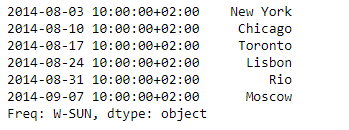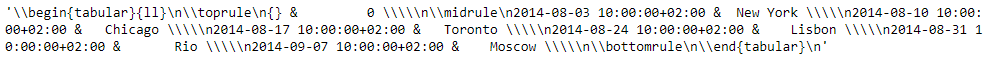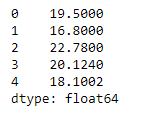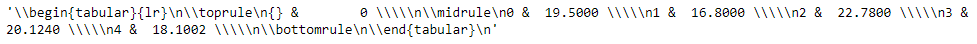# Python | Pandas Series.to_latex()

Pandas series is a One-dimensional ndarray with axis labels. The labels need not be unique but must be a hashable type. The object supports both integer- and label-based indexing and provides a host of methods for performing operations involving the index.

Pandas` Series.to_latex()` function render an object to a LaTeX tabular environment table.

Syntax: Series.to_latex(buf=None, columns=None, col_space=None, header=True, index=True, na_rep=’NaN’, formatters=None, float_format=None, sparsify=None, index_names=True, bold_rows=False, column_format=None, longtable=None, escape=None, encoding=None, decimal=’.’, multicolumn=None, multicolumn_format=None, multirow=None)

Parameter :
buf : Buffer to write to. If None, the output is returned as a string.
columns : The subset of columns to write. Writes all columns by default.
col_space : The minimum width of each column.
header : Write out the column names. If a list of strings is given, it is assumed to be aliases for the column names.
index : Write row names (index)
na_rep : Missing data representation.
formatters : Formatter functions to apply to columns’ elements by position or name.
float_format : Format string for floating point numbers.
sparsify : Set to False for a DataFrame with a hierarchical index to print every multi-index key at each row.

Returns : If buf is None, returns the resulting LateX format as a string, otherwise, returns None.

Example #1: Use `Series.to_latex()` function to render the given series object to LaTeX tabular enviornment table.

 `# importing pandas as pd ` `import` `pandas as pd ` ` `  `# Creating the Series ` `sr ``=` `pd.Series([``'New York'``, ``'Chicago'``, ``'Toronto'``, ``'Lisbon'``, ``'Rio'``, ``'Moscow'``]) ` ` `  `# Create the Datetime Index ` `didx ``=` `pd.DatetimeIndex(start ``=``'2014-08-01 10:00'``, freq ``=``'W'``,  ` `                     ``periods ``=` `6``, tz ``=` `'Europe/Berlin'``)  ` ` `  `# set the index ` `sr.index ``=` `didx ` ` `  `# Print the series ` `print``(sr) `

Output :Now we will use `Series.to_latex()` function to render the given series object to LaTeX tabular enviornment table.

 `# render to LaTeX tabular enviornment table. ` `sr.to_latex() `

Output :As we can see in the output, the `Series.to_latex()` function has successfully rendered the given series object to LaTeX tabular enviornment table.

Example #2: Use `Series.to_latex()` function to render the given series object to LaTeX tabular enviornment table.

 `# importing pandas as pd ` `import` `pandas as pd ` ` `  `# Creating the Series ` `sr ``=` `pd.Series([``19.5``, ``16.8``, ``22.78``, ``20.124``, ``18.1002``]) ` ` `  `# Print the series ` `print``(sr) `

Output :Now we will use `Series.to_latex()` function to render the given series object to LaTeX tabular enviornment table.

 `# render to LaTeX tabular enviornment table. ` `sr.to_latex() `

Output :As we can see in the output, the `Series.to_latex()` function has successfully rendered the given series object to LaTeX tabular enviornment table.

My Personal Notes arrow_drop_upCheck out this Author's contributed articles.

If you like GeeksforGeeks and would like to contribute, you can also write an article using contribute.geeksforgeeks.org or mail your article to contribute@geeksforgeeks.org. See your article appearing on the GeeksforGeeks main page and help other Geeks.

Please Improve this article if you find anything incorrect by clicking on the "Improve Article" button below.

Article Tags :

Be the First to upvote.

Please write to us at contribute@geeksforgeeks.org to report any issue with the above content.